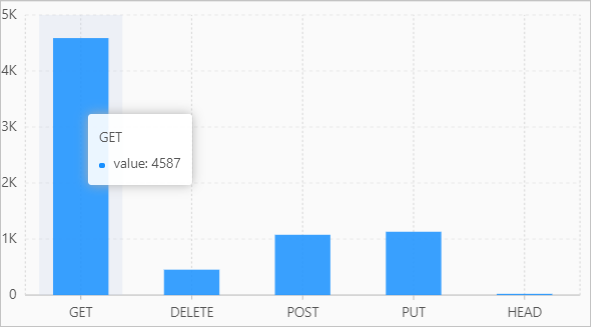## 语法

• 将array类型的数据展开为多行单列形式，列名为column_name
``UNNEST(x) AS table_alias(column_name)``
• 将map类型的数据展开为多行多列形式，列名为key_namevalue_name
``UNNEST(y) AS table(key_name,value_name)``

## 参数说明

x 数据类型为array类型。
column_name 将array类型的数据展开后，指定一个列名。该列用于存放array中的元素。
y 数据类型为map类型。
key_name 将map类型的数据展开后，指定一个列名。该列用于存放map中的键。
value_name 将map类型的数据展开后，指定一个列名。该列用于存放map中的键值。

## 示例

### 示例1

number字段的值（array类型）展开为多行单列形式。

• 字段样例
``number:[49, 50, 45, 47, 50]``
• 查询和分析语句
``````* |
SELECT
a
FROM  log,
UNNEST(cast(json_parse(number) AS array(bigint))) AS t(a)``````
• 查询和分析结果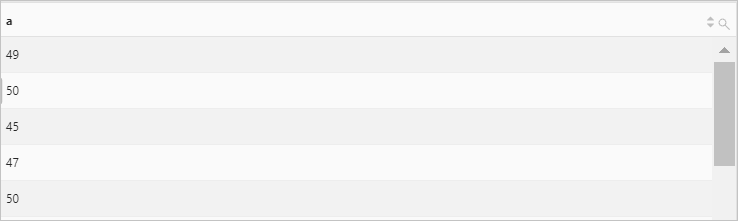### 示例2

number字段的值（array类型）展开为多行单列形式，并进行求和计算。

• 字段样例

此处仅提供一条日志样例，求和计算是针对所有日志，即对所有日志中的number字段的值进行求和。

``number:[49, 50, 45, 47, 50]``
• 查询和分析语句
``````* |
SELECT
sum(a) AS sum
FROM  log,
UNNEST(cast(json_parse(number) as array(bigint))) AS t(a)``````
• 查询和分析结果### 示例3

number字段的值（array类型）展开为多行单列形式，并对各个值进行分组统计。

• 字段样例
``number:[49, 50, 45, 47, 50]``
• 查询和分析语句
``````* |
SELECT
a, count(*) AS count
FROM  log,
UNNEST(cast(json_parse(number) as array(bigint))) AS t(a) GROUP BY a``````
• 查询和分析结果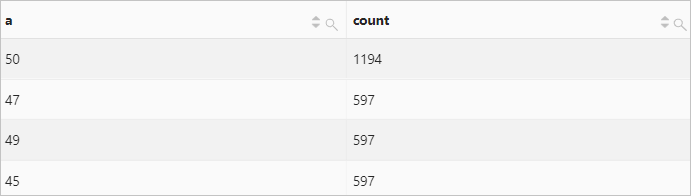### 示例4

number字段的值（map类型）展开为多行多列形式。

• 字段样例
``````result:{
anomaly_type:"OverThreshold"
dim_name:"request_time"
is_anomaly:true
score:1
value:"3.000000"}``````
• 查询和分析语句
``````* |
select
key,
value
FROM  log,
UNNEST(
try_cast(json_parse(result) as map(varchar, varchar))
) AS t(key, value)``````
• 查询和分析结果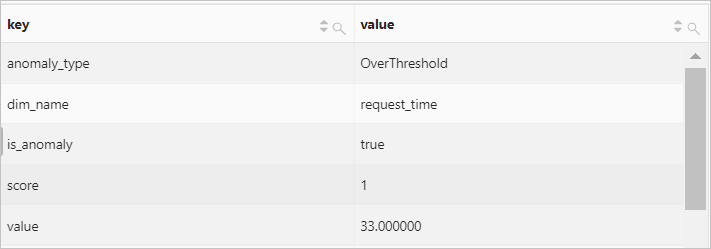### 示例5

number字段的值（map类型）展开为多行多列形式，并对各个键进行分组统计。

• 字段样例
``````result:{
anomaly_type:"OverThreshold"
dim_name:"request_time"
is_anomaly:true
score:1
value:"3.000000"}``````
• 查询和分析语句
``````* |
select
key,
count(*) AS count
FROM  log,
UNNEST(
try_cast(json_parse(result) as map(varchar, varchar))
) AS t(key, value)
GROUP BY
key``````
• 查询和分析结果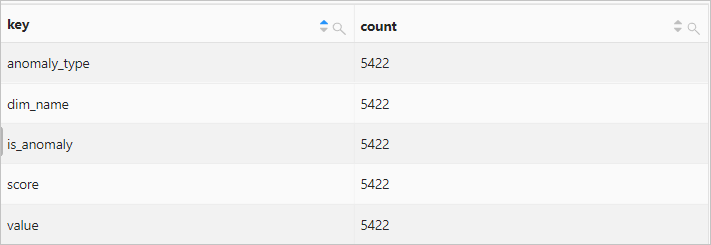### 示例6

• 查询和分析语句
``````* |
SELECT
key,
value
FROM(
SELECT
histogram(request_method) AS result
FROM      log
),
UNNEST(result) AS t(key, value)``````
• 查询和分析结果